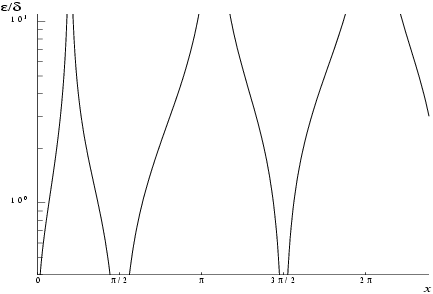NAG Library Function Document

1Purpose

nag_cos_integral (s13acc) returns the value of the cosine integral
 $Cix=γ+ln⁡x+∫0xcos⁡u-1udu, x>0$
where $\gamma$ denotes Euler's constant.

2Specification

 #include #include
 double nag_cos_integral (double x, NagError *fail)

3Description

nag_cos_integral (s13acc) calculates an approximate value for $\mathrm{Ci}\left(x\right)$.
For $0 it is based on the Chebyshev expansion
 $Cix=ln⁡x+∑r=0′arTrt,t=2 x16 2-1.$
For $16 where the value of ${x}_{\mathrm{hi}}$ is given in the Users' Note for your implementation,
 $Cix=fxsin⁡xx-gxcos⁡xx2$
where $f\left(x\right)=\underset{r=0}{{\sum }^{\prime }}\phantom{\rule{0.25em}{0ex}}{f}_{r}{T}_{r}\left(t\right)$ and $g\left(x\right)=\underset{r=0}{{\sum }^{\prime }}\phantom{\rule{0.25em}{0ex}}{g}_{r}{T}_{r}\left(t\right)$, $t=2{\left(\frac{16}{x}\right)}^{2}-1$.
For $x\ge {x}_{\mathrm{hi}}$, $\mathrm{Ci}\left(x\right)=0$ to within the accuracy possible (see Section 7).
Abramowitz M and Stegun I A (1972) Handbook of Mathematical Functions (3rd Edition) Dover Publications

5Arguments

1:    $\mathbf{x}$doubleInput
On entry: the argument $x$ of the function.
Constraint: ${\mathbf{x}}>0.0$.
2:    $\mathbf{fail}$NagError *Input/Output
The NAG error argument (see Section 3.7 in How to Use the NAG Library and its Documentation).

6Error Indicators and Warnings

NE_ALLOC_FAIL
Dynamic memory allocation failed.
See Section 2.3.1.2 in How to Use the NAG Library and its Documentation for further information.
NE_INTERNAL_ERROR
An internal error has occurred in this function. Check the function call and any array sizes. If the call is correct then please contact NAG for assistance.
See Section 2.7.6 in How to Use the NAG Library and its Documentation for further information.
NE_NO_LICENCE
Your licence key may have expired or may not have been installed correctly.
See Section 2.7.5 in How to Use the NAG Library and its Documentation for further information.
NE_REAL_ARG_LE
On entry, ${\mathbf{x}}=〈\mathit{\text{value}}〉$.
Constraint: ${\mathbf{x}}>0.0$.
The function has been called with an argument less than or equal to zero for which $\mathrm{Ci}\left(x\right)$ is not defined.

7Accuracy

If $E$ and $\epsilon$ are the absolute and relative errors in the result and $\delta$ is the relative error in the argument then in principle these are related by
 $E≃ δ cos⁡x and ​ ε≃ δ cos⁡x Cix .$
That is accuracy will be limited by machine precision near the origin and near the zeros of $\mathrm{cos}x$, but near the zeros of $\mathrm{Ci}\left(x\right)$ only absolute accuracy can be maintained.
The behaviour of this amplification is shown in Figure 1.Figure 1
For large values of $x$, $\mathrm{Ci}\left(x\right)\sim \frac{\mathrm{sin}x}{x}$ therefore $\epsilon \sim \delta x\mathrm{cot}x$ and since $\delta$ is limited by the finite precision of the machine it becomes impossible to return results which have any relative accuracy. That is, when $x\ge 1/\delta$ we have that $\left|\mathrm{Ci}\left(x\right)\right|\le 1/x\sim E$ and hence is not significantly different from zero.
Hence ${x}_{\mathrm{hi}}$ is chosen such that for values of $x\ge {x}_{\mathrm{hi}}$, $\mathrm{Ci}\left(x\right)$ in principle would have values less than the machine precision and so is essentially zero.

8Parallelism and Performance

nag_cos_integral (s13acc) is not threaded in any implementation.

None.

10Example

This example reads values of the argument $x$ from a file, evaluates the function at each value of $x$ and prints the results.

10.1Program Text

Program Text (s13acce.c)

10.2Program Data

Program Data (s13acce.d)

10.3Program Results

Program Results (s13acce.r)

© The Numerical Algorithms Group Ltd, Oxford, UK. 2017